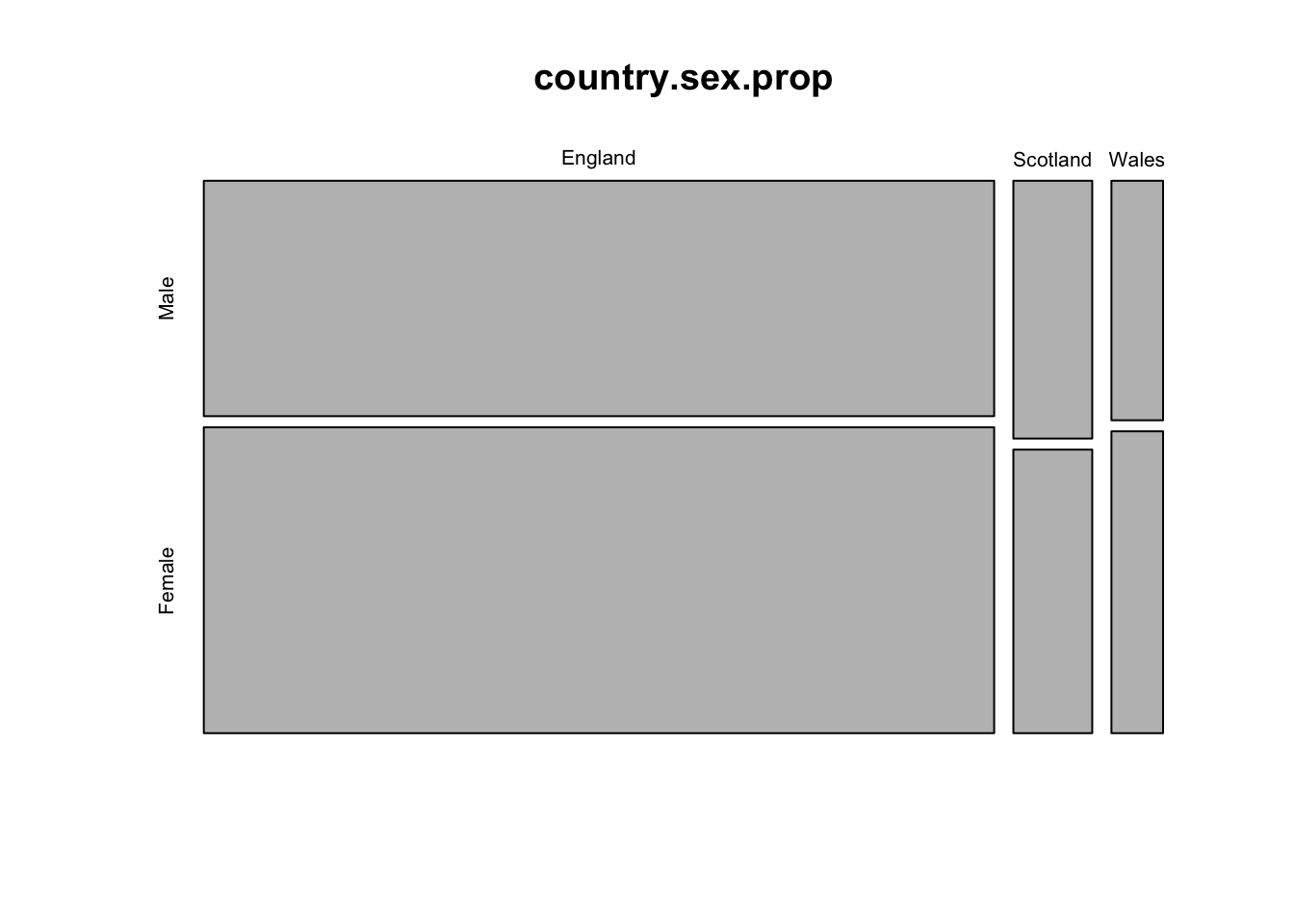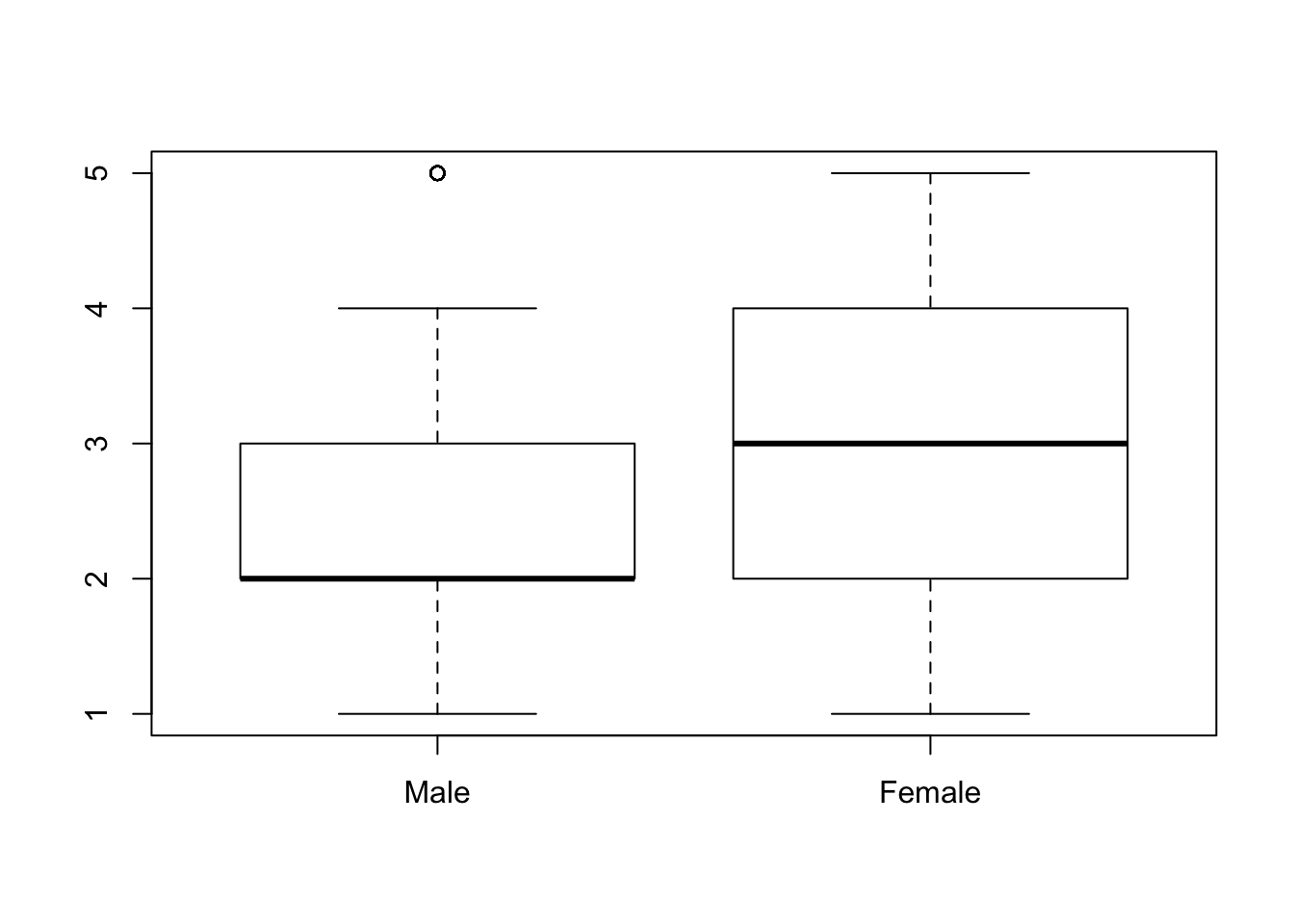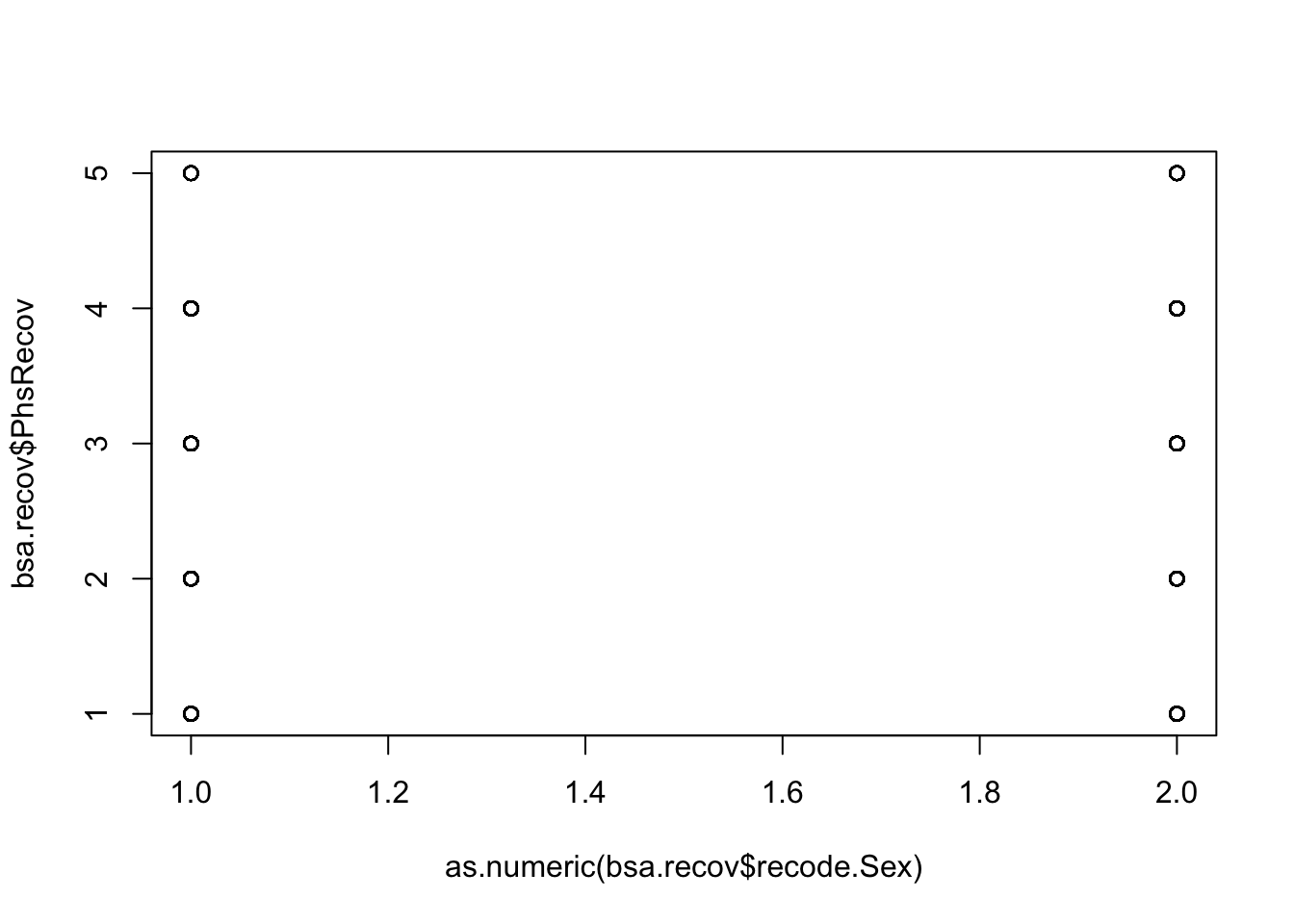# Outline

We’ve covered the basics of bivariate regression. Now it is time to tackle real data.

# British Social Attitudes

The BSA is a rich data source and available here. A copy of the BSA is here. There are technical details available which outline how the survey was carried out. The user guide is useful, too.

The technical notes also - and very usefully, what nice people! - offer guidance on statistical analysis of the data. The relevent section is at the bottom of the document and is written in readable text. What a relief!

Please download the tab data file containing the BSA responses. You may need to fill in some deails and login using Athens before you can access the data.

Tab data files are an open format. Each row is on a single line of the file. Columns are seperated by a tab. We can load this data in R using the read.table command. Note, we specify the data having headers and that columns are seperated by the tab character ‘’.

``````rm(list=ls()) # clear the enviroment

## Getting a handle on things

The data set is quite large. We can see exactly how large using ncol and nrow.

``ncol(bsa)``
``##  822``
``nrow(bsa)``
``##  2942``

We should probably not try to list all 822 variables at once. Instead, we can look at the documentation. In the documentation the variable name is given before the question.

R has quite a nice function for looking at the frequency of values called table. We can look at how many people identify as male and female.

``table(bsa\$Rsex)``
``````##
##    1    2
## 1291 1651``````

These are actually male and female! Which is which? As we can see these values are integers.

``head(bsa\$Rsex)``
``##  2 1 1 2 2 1``
``str(bsa\$Rsex)``
``##  int [1:2942] 2 1 1 2 2 1 1 2 1 2 ...``

If we check the user guide then we learn that 1 is Male and 2 is Female. To make our life a little easier we can change these 1s and 2s to categories of Male and Female. The factor function is perfect for this.

``````# Create new factor column in data based on value of Rsex
bsa\$recode.Sex <- factor(x = bsa\$Rsex, labels = c('Male', 'Female'))

str(bsa\$recode.Sex)``````
``##  Factor w/ 2 levels "Male","Female": 2 1 1 2 2 1 1 2 1 2 ...``
``````# we can check and make sure it works

``````##  Female Male   Male   Female Female Male
## Levels: Male Female``````
``head(bsa\$Rsex)``
``##  2 1 1 2 2 1``
``table(bsa\$recode.Sex)``
``````##
##   Male Female
##   1291   1651``````

That makes more sense. A quick note for later, for a regression the outcome should be ints or numeric; predictors can be factors with two levels (e.g., Male and Female). For predictors with more than two levels use dummy variables (you may be introduced to these later in your course).

Similiarly, we can change country to factor too.

``````bsa\$recode.Country <- factor(x = bsa\$Country, labels = c('England', 'Scotland', 'Wales'))
``````##  England England England England England England
## Levels: England Scotland Wales``````
``head(bsa\$Country)``
``##  1 1 1 1 1 1``

We can use table to create frequency table of our different variables. Let’s consider how many of each sex we have in each country.

``table(bsa\$recode.Country, bsa\$recode.Sex)``
``````##
##            Male Female
##   England  1098   1427
##   Scotland  120    132
##   Wales      73     92``````

There are more samples from England - as one might expect. What is perhaps surprising is that there are about 1/3 more females than males in England. Why do you think this is?

We can look at this more clearly as proportion of the total samples.

``````x <- table(bsa\$recode.Country, bsa\$recode.Sex)
country.sex.prop <- x/sum(x)
country.sex.prop``````
``````##
##                  Male     Female
##   England  0.37321550 0.48504419
##   Scotland 0.04078858 0.04486744
##   Wales    0.02481305 0.03127124``````

Which we can round to two decimal places for aesthetic reasons.

``round(country.sex.prop, digits = 2)``
``````##
##            Male Female
##   England  0.37   0.49
##   Scotland 0.04   0.04
##   Wales    0.02   0.03``````

The plot functions can produce different plots depending on the data type of the object you are passing to it. For example, country.sex.prop is a table type.

``class(country.sex.prop)``
``##  "table"``

Plotting a table type gives you quite a nice tile plot.

``plot(country.sex.prop)``But that might not be useful. In this case a table would probably communicate your point more clearly. What do you think?

Making variables into factors lets us select subsets of the data quite easily and with readable code.

``bsa.england <- bsa[bsa\$recode.Country == 'England',]``

We can also select specific combination of groups.

``bsa.england_female <- bsa[bsa\$recode.Country == 'England' && bsa\$recode.Sex == 'Female',]``

To select these data I created a logical index. For example, bsa\$recode.Country == ‘England’ creates a series of TRUE and FALSE values. If the country is England then the value is TRUE and the value if FALSE when the country is something other than England. We can check this too.

``table(bsa\$recode.Country == 'England')``
``````##
## FALSE  TRUE
##   417  2525``````

Indexing bsa with this lets us get only those rows where the index is TRUE. We index a data frame using []. So, this gives us all the data where country is England.

``bsa.england <- bsa[bsa\$recode.Country == 'England',]``

We can change other responses to factors for readability.

``````bsa\$recode.ChildHh <- factor(bsa\$ChildHh, levels = c(1,2,8,9), labels = c('Yes','No', "(Don't know)", '(Refusal)') )

# Do you normally read any daily morning newspaper at least 3 times a week?
bsa\$recode.Readpap <-  factor(bsa\$Readpap, levels = c(1,2,8,9), labels = c('Yes', 'No', "(Don't know)", '(Refusal)'))

# How much interest do you generally have in what is going on in politics?
bsa\$recode.Politics <- factor(bsa\$Politics, levels = c(1,2,3,4,5,8,9), labels = c('a great deal', 'quite a lot', 'some', 'not very much', 'none at all?', "(Don't know)", '(Refusal)'))``````

### Group work

Can you table some of these variables? Try producing some of those tile plots. Have a look at the questions. Do you think there are any interesting hypothesis you would like to investigate? Note the variable names; they will come in useful later.

Note: To keep the next section simple, try to pick predictors which are likert scales or two level factors (categories with only two values) and outcomes which are likert scales.

## Hypothesis?

We have some predictors from above:

1. Sex
2. Household Children
3. Morning newspapers read or not
4. Interest in politics

The BSA has a considerable number of questions. Using regressions we can try and see if one of these predictors is a significant predictor of a response.

Answering these questions for us is quite straight forward. We will pick a predictor and an outcome using an interesting hypothesis. Then we plot the data, fit a model and see if there is a significant trend.

Note We have intentionally chosen outcome variables on a likert scale. A likert scale is a response such as strongly agree(1), agree(2), neither agree or disagree(3), disagree(4), strongly disagree(5). We can assume there is an ordering to these responses where 1<2<3<4<5. For demonstration purposes we will consider these to be continious variables. However, some argue that the distance between 1-2 may not be the same as 2-3. An alternative way to analyse this data is an ordinal logistic regression. Logistic regression is beyond this session so we will focus on bivariate regression.

### Round one

1. Men [recode.Sex] are more likely than women to think that going to work will improve back problems which are starting to heal[PhsRecov].

And thinking of this same person who has been off work from their office job with a back problem, but is starting to feel better. How strongly do you agree or disagree that, in principle, going back to work quickly will help speed their recovery?

Our predictor is recode.Sex and our outcome is PhsRecov. The documentation gives PhsRecov as

[PhsRecov] CARD B13 And thinking of this same person who has been off work from their office job with a back problem, but is starting to feel better. How strongly do you agree or disagree that, in principle, going back to work quickly will help speed their recovery? 1 Agree strongly 2 Agree 3 Neither agree nor disagree 4 Disagree 5 Disagree strongly 8 (Don’t know) 9 (Refusal)

We are going to consider only those people who gave a 1 to 5 response. The reason for this is that we get to keep a nice likert scale. We can subset this data as so:

``````bsa.recov <- bsa[bsa\$PhsRecov < 6,]

# let us check to make sure all our chosen responses are less than 6
table(bsa.recov\$PhsRecov)``````
``````##
##    1    2    3    4    5
##  269 1092  894  578   86``````

We are not going to convert our outcome to a factor. This is because our outcome needs to be a continious variable (a number).

Let us look at our data.

``plot(bsa.recov\$recode.Sex, bsa.recov\$PhsRecov)``This is a boxplot. This type of visualisation is useful as it shows us where most of our data is. The thick black line is the median - where most of our data is - and the box is where 95% of our data is. We see that men generally tend to give a response of 2 - they agree that you should go back to work if your back has recovered a little. Women, on the other hand, tend to neither agree or disagree.

If you prefer to see the raw data instead of the boxplot, then convert recode.Sex to a number before plotting.

``plot(as.numeric(bsa.recov\$recode.Sex), bsa.recov\$PhsRecov)``We have lots of data and responses of either 1, 2, 3, 4 or 5. Moving the points might be more useful.

``plot(jitter(as.numeric(bsa.recov\$recode.Sex), factor=0.5), bsa.recov\$PhsRecov)``